# Flutter - Dart 3α 新特性 Record 和 Patterns 的提前預覽講解

## theme: smartblue

Record 和 Patterns 作為 Dart 3 的 Big Things ，無疑是 Flutter 和 Dart 開發者都十分關注的新特性。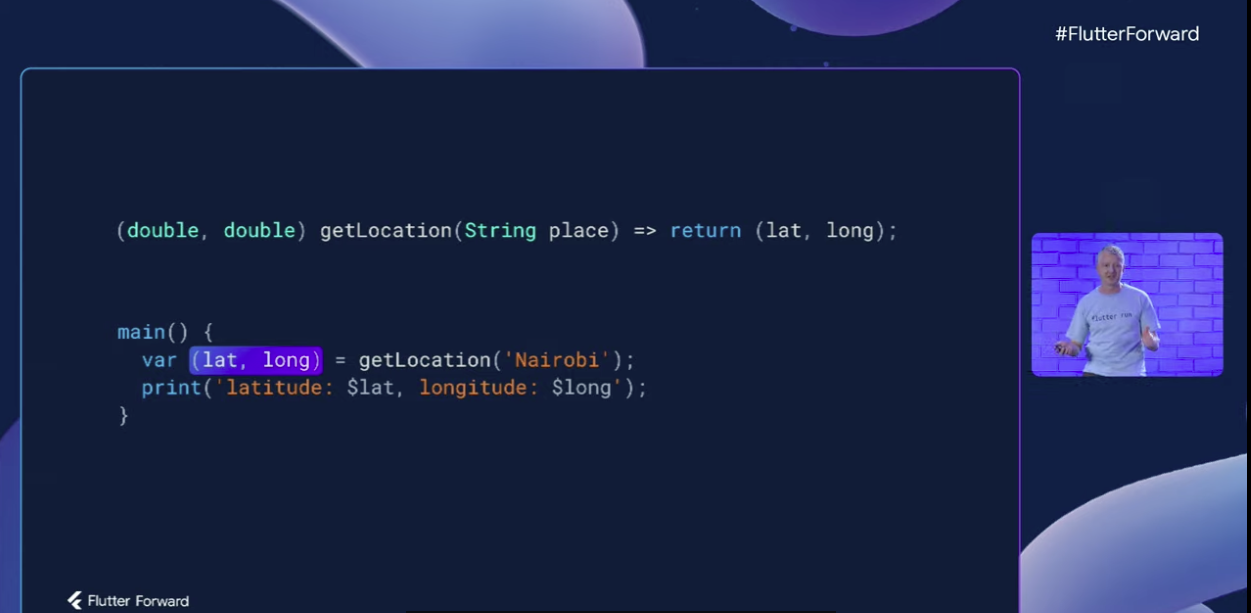# Records

```dart var record = (1, a: 2, 3, b: 4);```

• 定義了類，也就是說你的資料集合需要和特定類耦合
• 使用 Records 就不必宣告對應型別，只要具有相同欄位集的記錄， Dart 就會認為它們是相同型別（這個後面會介紹）

## 簡單介紹

```dart var record = (1, a: 2, 3, b: 4); print(record.\$1); // Print "1" print(record.a); // Print "2" print(record.\$2); // Print "3" print(record.b); // Print "4"```

```dart var num = (123); // num var records = (123,); // record```

• 如果沒有 "`,`" ，那麼 `(123)` 就是一個 num 型別的物件
• 有 "`,`" 之後 `(123,)` 才會被識別為是一個 Records 型別

```dart (bool, num, {int n, String s}) records; records = (false, 1, n: 12, s : "xxx"); print(records);```

```dart records = (false, 1, s : "xxx2"); records = (false, 1, n : 12);```

```dart records = (false, 1, s : "xxx2", n : 12); records = (s : "xxx2", n : 12, false, 1, ); print(records);```

• 同一命名欄位名稱只能出現一次，這個不難理解，比如上面程式碼你不可能定義兩個 `s`
• `(,)` 這樣的表示式是不允許的，但是 `()` 可以是沒有任何欄位的常量空 Records
• 有引數但是隻有 `()` 沒有 "`,`" 也不是 Records ，如 `(6)`
• 命令為 `hashCode``runtimeType``noSuchMethod`, 、`toString` 的欄位是不允許的
• 以下劃線開頭的命令欄位是不允許的
• 與位置欄位名稱衝突的命令欄位，比如 `('pos', \$1: 'named')` 這樣是不行的，但是 `(\$1: 'records')` 這樣可以

```dart var t = (int, String); print(t); print(t.\$0.runtimeType); print(t.\$1.runtimeType);```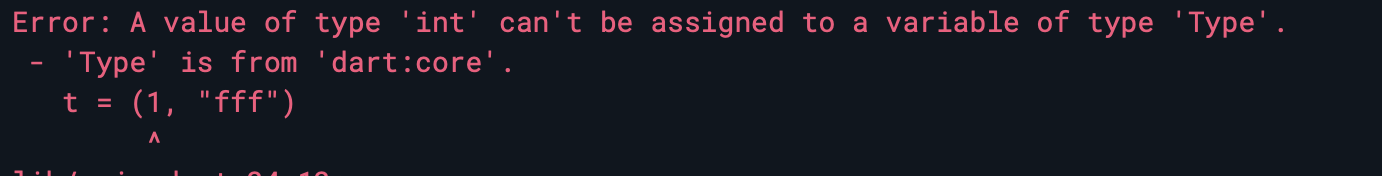• `Record``Object``dynamic` 的子類和 `Never` 的父類
• 所有的 Records 都是 `Record` 的子類和 `Never` 的父類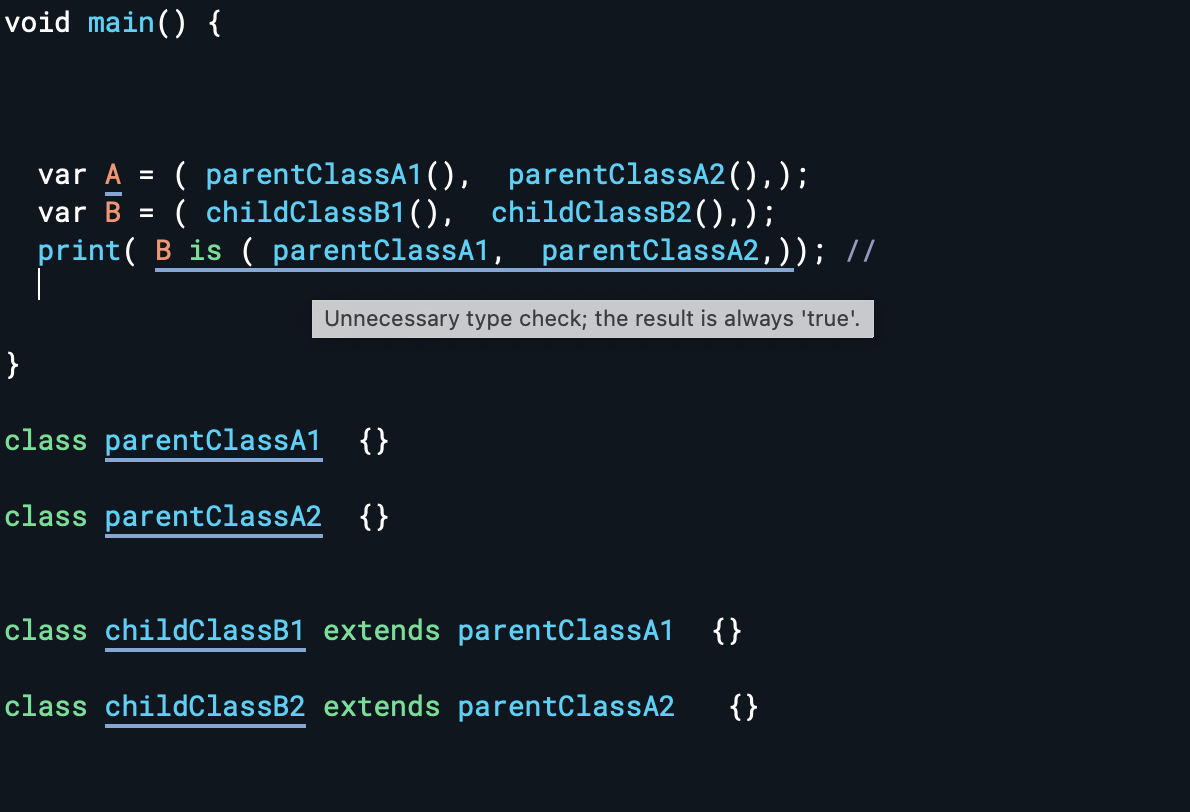## 進階探索

```dart class extends Record { double get \$1; String get name; bool get \$2; int get count; }```

Records 裡每個欄位都有 getter ，並且欄位是不可變的，所以不會又 Setter

```dart print((a: 1, b: 2) == (b: 2, a: 1)); // true```

```dart print((true, 2, a: 1, b: 2,) == (2, true, b: 2, a: 1)); // false```

```dart (num, Object) pair = (1, 2.3); print(pair is (int, double)); // "true".```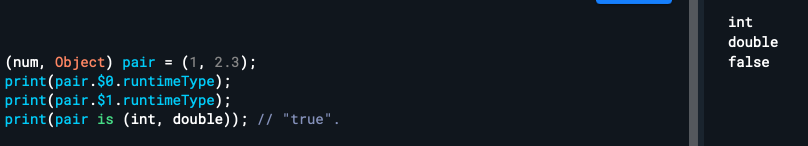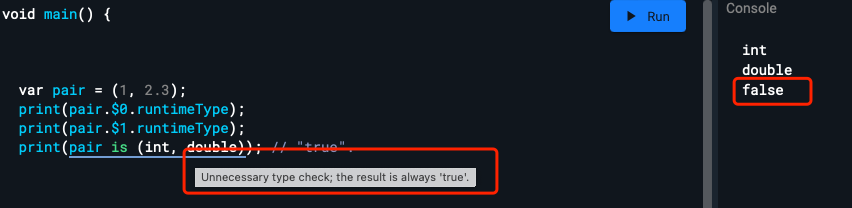```dart var map = {}; map[(1, "aa")] = "value"; print(map[(1, "aa")]); //輸出 "value"```

```dart

class newClass {

}

var map = {}; map[(1, new newClass())] = "value"; print(map[(1, new newClass())]); //輸出 "null"

```

```dart class newClass {

@override bool operator ==(Object other) { return true; }

@override int get hashCode => 1111111;

} ```

```dart int say(int i) { print(i); return i; }

var x = (a: say(1), b: say(2)); var y = (b: say(3), a: say(4));

```

```dart var x = (say(0), a: say(1), b: say(2)); var y = (b: say(3), a: say(4), say(5));```

## Records 帶來的語法歧義

### try/on

```dart void recordTryOn() { try { } on String { }

``````on(int, String) {
}
``````

} ```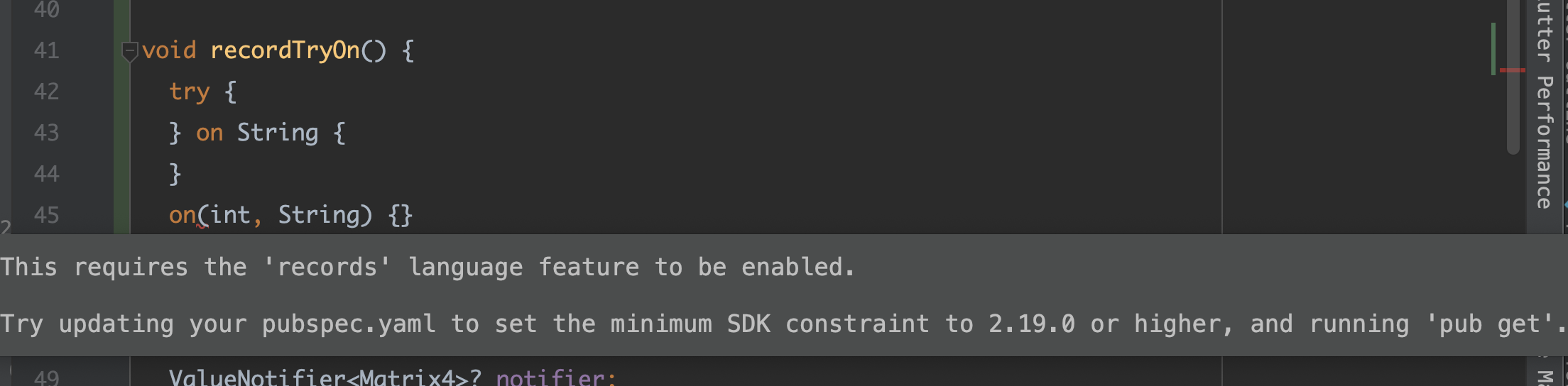```dart @metadata (a, b) function() {}```

• `@metadata(a, b)` 與沒有返回型別的函式宣告關聯的metadata 註解
• `@metadata`與返回型別為 Records 型別的函式關聯的metadata 註解 `(a, b)`

@metadata (a, b) function() {} ```

• 前者由於 `@metadata` 之後沒有空格，所以表示為 `(a, b)` 的 metadata 註解
• 前者由於有空格，所以表示為 Records 返回型別

@metadata // Comment. (x,) a; ```

```dart @TestMeta(1, "2") class C {}

class TestMeta { final String message; final num code;

const TestMeta(this.code, this.message);

@override String toString() => "feature: \$code, \$message"; } ```

```dart @TestMeta (1, "2") //Error class C {}```

### toString

```print((1, 2, 3).toString()); // "(1, 2, 3)". print((a: 'str', 'int').toString()); // "(a: str, int)".```

Debug 版本中，命名欄位出現的順序以及它們如何與位置欄位進行排列是不確定的，只有位置欄位必須按位置順序出現

# Patterns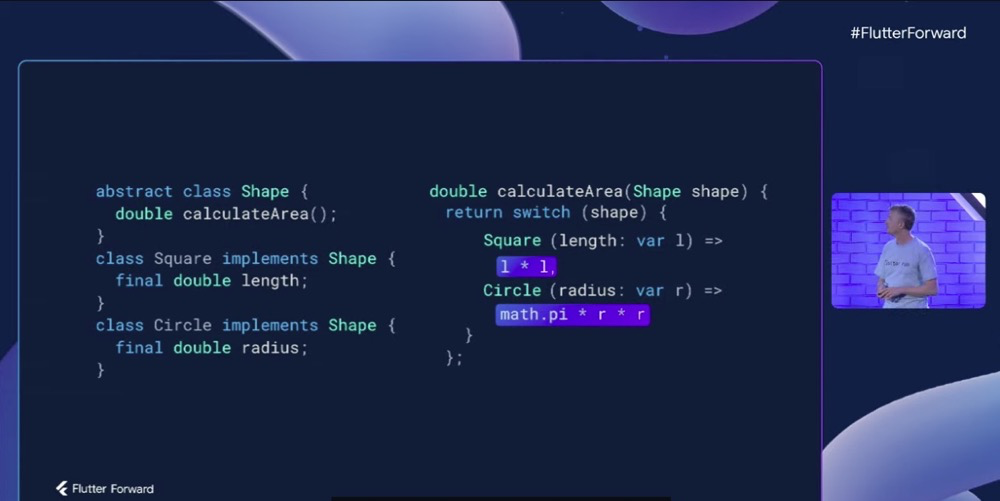## 簡單介紹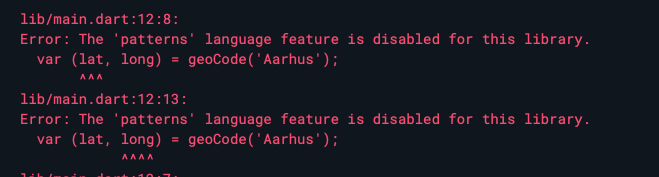### 多返回值

```dart (double, double) geoCode(String city) { var lat = // Calculate... var long = // Calculate...

return (lat, long); // Wrap in record and return. } ```

```dart var (lat, long) = geoCode('Aarhus'); print('Location lat:\$lat, long:\$long');```

```dart var list = [1, 2, 3]; var [a, b, c] = list; print(a + b + c); // 6.

var map = {'first': 1, 'second': 2}; var {'first': a, 'second': b} = map; print(a + b); // 3. ```

```dart var (a, b) = ('left', 'right'); (b, a) = (a, b); // Swap! print('\$a \$b'); // Prints "right left".```

### 代數資料型別

```dart ///before double calculateArea(Shape shape) { if (shape is Square) { return shape.length + shape.length; } else if (shape is Circle) { return math.pi * shape.radius * shape.radius; } else { throw ArgumentError("Unexpected shape."); } }

//after double calculateArea(Shape shape) => switch (shape) { Square(length: var l) => l * l, Circle(radius: var r) => math.pi * r * r }; ```

### Patterns

```dart var isPrimary = switch (color) { Color.red || Color.yellow || Color.blue => true, _ => false };```

`"||"`可以在 switch 中讓多個 case 共享一個主體，`"_"` 表示預設，甚至如下程式碼所示，你還可以在繫結 `s` 之後，多個共享一個 `when` 條件：

```dart switch (shape) { case Square(size: var s) || Circle(size: var s) when s > 0: print('Non-empty symmetric shape'); case Square() || Circle(): print('Empty symmetric shape'); default: print('Asymmetric shape'); }```

```dart String asciiCharType(int char) { const space = 32; const zero = 48; const nine = 57;

return switch (char) { < space => 'control', == space => 'space', > space && < zero => 'punctuation', >= zero && <= nine => 'digit' // Etc... } } ```

```dart String? maybeString = ... switch (maybeString) { case var s?: // s has type non-nullable String here. }```

```dart (int?, int?) position = ...

// We know if we get here that the coordinates should be present: var (x!, y!) = position;

List row = ...

// If the first column is 'user', we expect to have a name after it. switch (row) { case ['user', var name!]: // name is a non-nullable string here. } ```

```dart switch ((1, 2)) { case (var a, var b): ... }

switch (record) { case (int x, String s): print('First field is int \$x and second is String \$s.'); } ``````dart var list = [1, 2, 3]; var [, two, ] = list;

var [a, b, ...rest, c, d] = [1, 2, 3, 4, 5, 6, 7]; print('\$a \$b \$rest \$c \$d'); // Prints "1 2 [3, 4, 5] 6 7".

// Variable: var (untyped: untyped, typed: int typed) = ... var (:untyped, :int typed) = ...

switch (obj) { case (untyped: var untyped, typed: int typed): ... case (:var untyped, :int typed): ... }

// Null-check and null-assert: switch (obj) { case (checked: var checked?, asserted: var asserted!): ... case (:var checked?, :var asserted!): ... }

// Cast: var (field: field as int) = ... var (:field as int) = ...

class Rect { final double width, height;

Rect(this.width, this.height); }

display(Object obj) { switch (obj) { case Rect(width: var w, height: var h): print('Rect \$w x \$h'); default: print(obj); } } ```